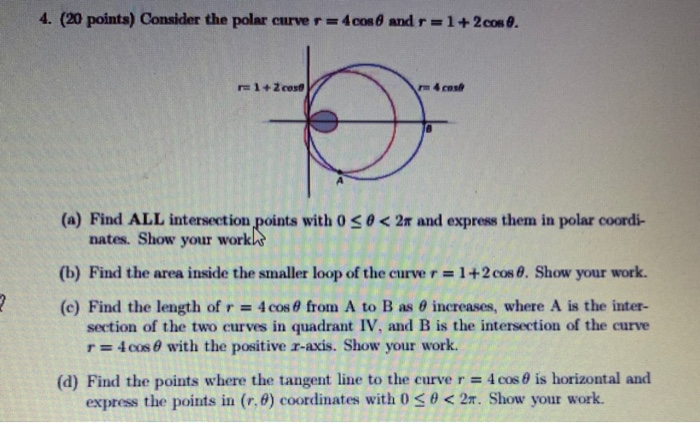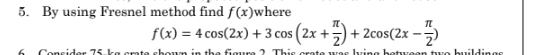# Prove: cos4x = 8cos^4x - 8cos^2x + 1 My Attempt: RS: = 4cos^2x (2cos^2x - 1) + 1 = 4 cos^2x (cos2x) + 1 LS: = cos2(2x) = 2cos^2(2x) - 1 = (cos^2(2)) - cos^2(2x)) - 1 ----- Prove: 8cos^4x = cos4x + 4cos2x + 3 My Attempt: RS: = cos2(2x) + 4cos(

Prove:
cos4x = 8cos^4x - 8cos^2x + 1

My Attempt:
RS:
= 4cos^2x (2cos^2x - 1) + 1
= 4 cos^2x (cos2x) + 1

LS:
= cos2(2x)
= 2cos^2(2x) - 1
= (cos^2(2)) - cos^2(2x)) - 1

-----

Prove:
8cos^4x = cos4x + 4cos2x + 3

My Attempt:
RS:
= cos2(2x) + 4cos(2x) + 3
= 2cos^2(2x) - 1 + 2(4)cos^2(2x) - 1 + 3

Do you mean... why isn't (cos(2x))^2 equal to cos(4x^2)?
= (4cos^2(x)-2)(2cos^2(x)-1)
= (8cos^4(x) + 2) (-4cos^2(x)+2)
verify identity
cos 2A sec A = 2cos A - sec A
Since you square the entire function, why is it (cos2x)(cos2x)? I mean, why don't you square the "2" into (cosx)(cos2x)?
All these numbers and things are getting confusing. Let's try to simplify this.

Could you tell me how you foiled this?
(4cos^2(x)-2)(2cos^2(x)-1)
No, it would be much harder. It's always easier to expand than to condense.

Expansion of the identities is a very important concept.

cos(2x) = 2cos^2(x) - 1
If it's cos(4x), then that's like cos(2*2x). Everything in the expansion is the same except the x will be 2x now, like 2cos^2(2x) - 1.

All you're doing is repeating the expansion process until you can't do it anymore.

If you have more questions that are confusing you, then I can try to help.
Whenever I try to prove the identity with the right side, I keep getting different answer

RS:
= cos2(2x) + 4(2cos^2(x)-1) + 3
= cos2(2x) + 8cos^2(x) - 4 + 3
= 2cos^2(2x) - 1 + 8cos^2(x) - 4 + 3
= 2cos^2(2x) + 8cos^2(x) - 2

...I think I'm making this too complicated than it should be...
Made a mistake on the last line

= (4cos(2x))*(2cos^2(2x) - 1)*(2cos^2(2x) - 1) * (cos(2x))
Ah okay, I get it now, so "2x" is like "x", they are always together

I'm working on the second question now and I'm expanding the identity, but I'm kind of stuck...

8cos^4x = cos4x + 4cos2x + 3

LS:
= 8cos^4x
= 8(cos^2x)(cos^2x)
= 4(cos^4(2x))
= 4(cos^2(2x))*(cos^2(2x))
= 4(cos2x)*(cos2x)*(cos2x)*(cos2x)
= (4cos^2(2x))*(2cos^2(2x) - 1)*(2cos^2(2x) - 1) * (cos(2x))
Ugh...nevermind I'm so confused...

If I did the right side, would it be more easy than the left side? I don't think I know how to expand with identities...
I'm working on it...
I'll try to explain the two lines better...

2cos^2(2x) - 1
This is like 2(cos^2(2x)) - 1.

In this line, we're changing only the cos^2(2x). When the squared is written on the "cos" part, that means the entire function is squared. Therefore, cos^2(2x) is the same as (cos(2x))^2.

When something is squared, you multiply it by itself. For example, 4^2 = 4*4, and (n+1)^2 = (n+1)(n+1).

Therefore, we are saying that (cos(2x))^2 = (cos(2x))(cos(2x)) = cos2x * cos2x. The rest of the line remained unchanged.
And where did you get the "- 2" from 2cos^2(2x) + 8cos^2(x) - 2
And I still get stuck...

= cos2(2x) + 4(2cos^2(2x)-1) + 3
= cos2(2x) + 8cos^2(2x) - 4 + 3
= 2cos^2(2x) - 1 + 8cos^2(2x) - 4 + 3
= 2cos^2(2x) + 8cos^2(2x) - 2

What am I doing wrong now?
Okay? But, I still don't get what you did in:

2cos^2(2x) - 1 Double-angle again.
2(cos2x * cos2x) - 1 Double-angle again.
##### Add Answer of: Prove: cos4x = 8cos^4x - 8cos^2x + 1 My Attempt: RS: = 4cos^2x (2cos^2x - 1) + 1 = 4 cos^2x (cos2x) + 1 LS: = cos2(2x) = 2cos^2(2x) - 1 = (cos^2(2)) - cos^2(2x)) - 1 ----- Prove: 8cos^4x = cos4x + 4cos2x + 3 My Attempt: RS: = cos2(2x) + 4cos(
Similar Homework Help Questions
• ### Prove: sin2x / 1 - cos2x = cotx My Attempt: LS: = 2sinxcosx / - 1 - (1 - 2sin^2x) = 2sinxcosx / - 1 + 2sin^2x = cosx / sinx - 1 = cosx / sinx - 1/1 = cosx / sinx - sinx / sinx -- Prove: 2sin(x+y)sin(x-y) = cos2y - cos2x My Attempt: RS: = 1 - 2sin^2

Prove:sin2x / 1 - cos2x = cotxMy Attempt:LS:= 2sinxcosx / - 1 - (1 - 2sin^2x)= 2sinxcosx / - 1 + 2sin^2x= cosx / sinx - 1= cosx / sinx - 1/1= cosx / sinx - sinx / sinx--Prove:2sin(x+y)sin(x-y) = cos2y - cos2xMy Attempt:RS:= 1 - 2sin^2y - 1 - 2sin^2x= 1 - 1 - 2sin^2y - 2sin^2x= -2sin^2y - 2sin^2x

• ### Prove the identity: 1-cos2x/sin2x = 1+tanx/1+cotx I simplified the RS to tanx

Prove the identity:1-cos2x/sin2x = 1+tanx/1+cotxI simplified the RS to tanx.LS:(1-2cos²x+1)/2sinxcosx(2+2cos²x)/2sinxcosxHow do I simplify the rest?

• ### 1) Verify the identity cos^4x - sin^4x = cos2x I know that this is the difference of squares so (cos^2x)^2 - (sin^2x)^2 is this correct for the first step

1) Verify the identity cos^4x - sin^4x = cos2x I know that this is the difference of squares so (cos^2x)^2 - (sin^2x)^2 is this correct for the first step?

• ### Solve the following equation for 0 less than and/or equal to "x" less than and/or equal to 360 -- cos^2x - 1 = sin^2x -- Attempt: cos^2x - 1 - sin^2x = 0 cos^2x - 1 - (1 - cos^2x) = 0 cos^2x - 1 - 1 + cos^2x = 0 2cos^2x - 2 = 0 (2cos^2x/2)= (-2/2) cos^

Solve the following equation for 0 less than and/or equal to "x" less than and/or equal to 360--cos^2x - 1 = sin^2x--Attempt:cos^2x - 1 - sin^2x = 0cos^2x - 1 - (1 - cos^2x) = 0cos^2x - 1 - 1 + cos^2x = 02cos^2x - 2 = 0(2cos^2x/2)= (-2/2)cos^2x = -1cosx = square root of -1And I can't do anything with this now...what am I doing wrong?--Textbook answers:0, 180, 360--

• ### 4. (20 points) Consider the polar curve r = 4cos 6 and r=1+2cos e. 1+2 caso...4. (20 points) Consider the polar curve r = 4cos 6 and r=1+2cos e. 1+2 caso (a) Find ALL intersection points with 0 So< 2x and express them in polar coordi- nates. Show your works (b) Find the area inside the smaller loop of the curve r = 1+2 cose. Show your work. (c) Find the length of r = 4 cose from A to B as 8 increases, where A is the inter- section of the two curves in...

• ### 5. By using Fresnel method find f(x)where f(x) = 4 cos(2x) + 3 cos (2x + 2) + 2cos(2-2) 6 Coneide...5. By using Fresnel method find f(x)where f(x) = 4 cos(2x) + 3 cos (2x + 2) + 2cos(2-2) 6 Coneider 75 re crte choum in the iouro2 Thie crnte I 5. By using Fresnel method find f(x)where f(x) = 4 cos(2x) + 3 cos (2x + 2) + 2cos(2-2) 6 Coneider 75 re crte choum in the iouro2 Thie crnte I

• ### The identity of: sin^4x+cos^4x= (sin^2x+cos^2x)(sin^2x+cos^2x) sin^2x+cos^2=1 This is the answer I come up with, it is not one of the options available as an answer

The identity of: sin^4x+cos^4x= (sin^2x+cos^2x)(sin^2x+cos^2x) sin^2x+cos^2=1 This is the answer I come up with, it is not one of the options available as an answer. The answers given are 1. -2sin^2xcos^2x 2. 1+2sin^2x-2sin^4x 3. 1+3sin^3x-2sin^2x 4. 1-2sin^2x+2sin^4x 5. 0

• ### verify those 3 identitiscsc x- cos x cot x = sin xsec 2x= sec^2x/2-sec^2xsin4x-sin3x/cos4x+ cos3x= 1-cos x/sinx

verify those 3 identitiscsc x- cos x cot x = sin xsec 2x= sec^2x/2-sec^2xsin4x-sin3x/cos4x+ cos3x= 1-cos x/sinx

• ### 1. a) Substitute u = sin(x) to evaluate sin^2(x) cos^3(x) dx. [trig identity sin2(x)+cos2(x) = 1]....

1. a) Substitute u = sin(x) to evaluate sin^2(x) cos^3(x) dx. [trig identity sin2(x)+cos2(x) = 1]. b) Find the antiderivatives: i) sin(2x) dx ii) (cos(4x)+3x^2) dx

• ### Prove this: #(1-sin^4x-cos^4x)/(1-sin^6x-cos^6x)=2/3#?

#(1-sin^4x-cos^4x)/(1-sin^6x-cos^6x)=2/3#

Free Homework App